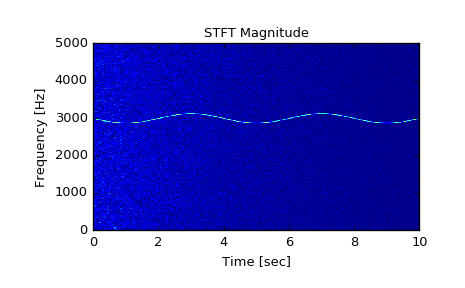# scipy.signal.stft¶

scipy.signal.stft(x, fs=1.0, window='hann', nperseg=256, noverlap=None, nfft=None, detrend=False, return_onesided=True, boundary='zeros', padded=True, axis=-1)[source]

Compute the Short Time Fourier Transform (STFT).

STFTs can be used as a way of quantifying the change of a nonstationary signal’s frequency and phase content over time.

Parameters: x : array_like Time series of measurement values fs : float, optional Sampling frequency of the x time series. Defaults to 1.0. window : str or tuple or array_like, optional Desired window to use. See get_window for a list of windows and required parameters. If window is array_like it will be used directly as the window and its length must be nperseg. Defaults to a Hann window. nperseg : int, optional Length of each segment. Defaults to 256. noverlap : int, optional Number of points to overlap between segments. If None, noverlap = nperseg // 2. Defaults to None. When specified, the COLA constraint must be met (see Notes below). nfft : int, optional Length of the FFT used, if a zero padded FFT is desired. If None, the FFT length is nperseg. Defaults to None. detrend : str or function or False, optional Specifies how to detrend each segment. If detrend is a string, it is passed as the type argument to the detrend function. If it is a function, it takes a segment and returns a detrended segment. If detrend is False, no detrending is done. Defaults to False. return_onesided : bool, optional If True, return a one-sided spectrum for real data. If False return a two-sided spectrum. Note that for complex data, a two-sided spectrum is always returned. Defaults to True. boundary : str or None, optional Specifies whether the input signal is extended at both ends, and how to generate the new values, in order to center the first windowed segment on the first input point. This has the benefit of enabling reconstruction of the first input point when the employed window function starts at zero. Valid options are ['even', 'odd', 'constant', 'zeros', None]. Defaults to ‘zeros’, for zero padding extension. I.e. [1, 2, 3, 4] is extended to [0, 1, 2, 3, 4, 0] for nperseg=3. padded : bool, optional Specifies whether the input signal is zero-padded at the end to make the signal fit exactly into an integer number of window segments, so that all of the signal is included in the output. Defaults to True. Padding occurs after boundary extension, if boundary is not None, and padded is True, as is the default. axis : int, optional Axis along which the STFT is computed; the default is over the last axis (i.e. axis=-1). f : ndarray Array of sample frequencies. t : ndarray Array of segment times. Zxx : ndarray STFT of x. By default, the last axis of Zxx corresponds to the segment times.

See also

istft
Inverse Short Time Fourier Transform
check_COLA
Check whether the Constant OverLap Add (COLA) constraint is met
welch
Power spectral density by Welch’s method.
spectrogram
Spectrogram by Welch’s method.
csd
Cross spectral density by Welch’s method.
lombscargle
Lomb-Scargle periodogram for unevenly sampled data

Notes

In order to enable inversion of an STFT via the inverse STFT in istft, the signal windowing must obey the constraint of “Constant OverLap Add” (COLA), and the input signal must have complete windowing coverage (i.e. (x.shape[axis] - nperseg) % (nperseg-noverlap) == 0). The padded argument may be used to accomplish this.

The COLA constraint ensures that every point in the input data is equally weighted, thereby avoiding aliasing and allowing full reconstruction. Whether a choice of window, nperseg, and noverlap satisfy this constraint can be tested with check_COLA.

New in version 0.19.0.

References

 [R265] Oppenheim, Alan V., Ronald W. Schafer, John R. Buck “Discrete-Time Signal Processing”, Prentice Hall, 1999.
 [R266] Daniel W. Griffin, Jae S. Limdt “Signal Estimation from Modified Short Fourier Transform”, IEEE 1984, 10.1109/TASSP.1984.1164317

Examples

>>> from scipy import signal
>>> import matplotlib.pyplot as plt


Generate a test signal, a 2 Vrms sine wave whose frequency is slowly modulated around 3kHz, corrupted by white noise of exponentially decreasing magnitude sampled at 10 kHz.

>>> fs = 10e3
>>> N = 1e5
>>> amp = 2 * np.sqrt(2)
>>> noise_power = 0.01 * fs / 2
>>> time = np.arange(N) / float(fs)
>>> mod = 500*np.cos(2*np.pi*0.25*time)
>>> carrier = amp * np.sin(2*np.pi*3e3*time + mod)
>>> noise = np.random.normal(scale=np.sqrt(noise_power),
...                          size=time.shape)
>>> noise *= np.exp(-time/5)
>>> x = carrier + noise


Compute and plot the STFT’s magnitude.

>>> f, t, Zxx = signal.stft(x, fs, nperseg=1000)
>>> plt.pcolormesh(t, f, np.abs(Zxx), vmin=0, vmax=amp)
>>> plt.title('STFT Magnitude')
>>> plt.ylabel('Frequency [Hz]')
>>> plt.xlabel('Time [sec]')
>>> plt.show()#### Previous topic

scipy.signal.vectorstrength

#### Next topic

scipy.signal.istft